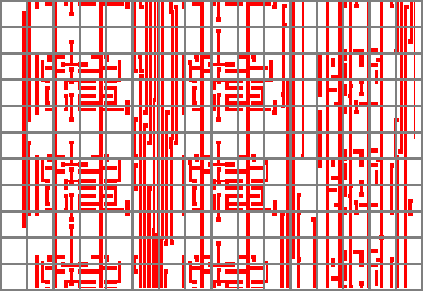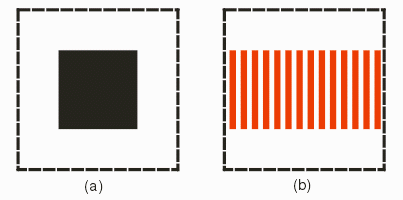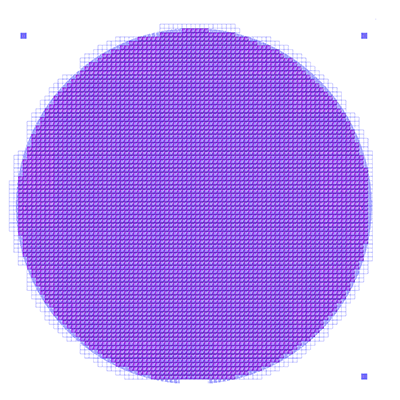# Area Fill - Metal Density Calculator

Area Fill is designed to calculate the area within a window that is covered by metal -- to be more specific, the area that is digitized. Knowing this area can be useful for calculations related to plating and to planarization (CMP).Figure 1 - A window (demo6.gds, layer=8) from -2946,2551 to -2742,2696 um. Metal Density?

The program clips the desired data to the window extents, unionizes the data (so that overlapping polygons do not contribute to an incorrect calculation) and then sums up the area of the results.

The output is written as an ASCII table.

### Boolean Layer Operations

Often, the metal pattern on the mask/wafer is defined not by a single GDSII data layer but rather by a combination of layers. Area Fill supports the union of layers, subtraction of one layer from another and reversal of layers.

### Tiling Area Calculations

Often one needs to calculate a matrix of local area or densities. For example, one may have a total field area of 25 x 25 mm but needs to know the metal density in 50 um increments. Area Fill has a tile argument that allows the user to define the tile size in X and Y or the number of tiles to use in X and Y.Figure 2 - Same window as Figure 1 but cut into small tiles. Each tile's density is calculated separately.

### Perimeter Calculations

It may be of interest to get a measure of the "spatial frequency" of a particular tile. Two tiles might have the same area fill value but tile (a) might have a single fat rectangle and tile (b) might be composed of many tightly spaced narrow lines.Figure 3 - Both tile (a) and (b) have identical area but are signficantly different.

Area Fill can give a single value indication of the frequency by calculating and reporting the x/y components of the conductor perimeters.

Area Fill breaks the view into tiles and for each tile we compute the x-perimeter and y-perimeter.

The perimeter of a polygon is the sum of lengths of the edges of that polygon. x-perimeter and y-perimeter are the same except that it's only along X and Y axis.

The sum of x perimeters divide by the sum of y-perimeters for all polygons in a tile is the x/y-perimeter of that tile - this information can be useful to the user.

X/YRelative is the ratio (in %) of the x/y-perimeter of a given tile to the maximum x/y-perimeter among all tiles.

The lengths of all edges in a tile are summed and grouped by angle (in the domain from 0 to 179 - angles are rounded to the nearest integer so there are 180 groups). The "dominant direction" corresponds to the group with the largest sum.

If `-falseperim` appears on the command line, then cutlines and butting edges are excluded from the calculation.

Perimeter Example

Click on link to see how AreaFill calculates the perimeter along X and Y axis.

Because Area Fill is often used for massive IC layout databases, the program can subdivide the computations using multiple threads. Our users routinely run jobs on 16 CPU's in a 64 bit Linux environment.

### Image Output

Some users might like to produce a density "image." The utility afill2tiff can covert the density (and perimeter) data into a TIFF image.

### Memory Footprint for OASIS files

When processing an OASIS file, user should have an idea how much RAM is needed in relation to the OASIS file size and nfork command line option. Details.

### Example File and Script

This web page explains the sample batch file we include in our examples folder. Example Detail.

### Metal Density for a Round Wafer?

Areafill only supports a rectangular window. A wafer is round. Can we still get the density of metal covering a wafer? Yes, go here to find out how.Figure 4 - How to get the density over a round wafer